## Taniyama-Shimura Conjecture

A conjecture which arose from several problems proposed by Taniyama in an international mathematics symposium in 1955. Let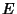be an Elliptic Curve whose equation has Integer Coefficients, let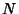be the Conductor ofand, for each, let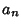be the number appearing in the-function of. Then there exists a Modular Form of weight two and levelwhich is an eigenform under the Hecke Operators and has a Fourier Series.

The conjecture says, in effect, that every rational Elliptic Curve is a Modular Form in disguise. Stated formally, the conjecture suggests that, for every Elliptic Curve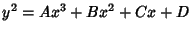over the Rationals, there exist nonconstant Modular Functionsandof the same levelsuch that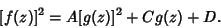Equivalently, for every Elliptic Curve, there is a Modular Form with the same Dirichlet L-Series.

In 1985, starting with a fictitious solution to Fermat's Last Theorem, G. Frey showed that he could create an unusual Elliptic Curve which appeared not to be modular. If the curve were not modular, then this would show that if Fermat's Last Theorem were false, then the Taniyama-Shimura conjecture would also be false. Furthermore, if the Taniyama-Shimura conjecture were true, then so would be Fermat's Last Theorem!

However, Frey did not actually prove whether his curve was modular. The conjecture that Frey's curve was modular came to be called the epsilon conjecture,'' and was quickly proved by Ribet (Ribet's Theorem) in 1986, establishing a very close link between two mathematical structures (the Taniyama-Shimura conjecture and Fermat's Last Theorem) which appeared previously to be completely unrelated.

As of the early 1990s, most mathematicians believed that the Taniyama-Shimura conjecture was not accessible to proof. However, A. Wiles was not one of these. He attempted to establish the correspondence between the set of Elliptic Curves and the set of modular elliptic curves by showing that the number of each was the same. Wiles accomplished this by counting'' Galois representations and comparing them with the number of modular forms. In 1993, after a monumental seven-year effort, Wiles (almost) proved the Taniyama-Shimura conjecture for special classes of curves called Semistable Elliptic Curves.

Wiles had tried to use horizontal Iwasawa theory to create a so-called Class Number formula, but was initially unsuccessful and therefore used instead an extension of a result of Flach based on ideas from Kolyvagin. However, there was a problem with this extension which was discovered during review of Wiles' manuscript in September 1993. Former student Richard Taylor came to Princeton in early 1994 to help Wiles patch up this error. After additional effort, Wiles discovered the reason that the Flach/Kolyvagin approach was failing, and also discovered that it was precisely what had prevented Iwasawa theory from working.

With this additional insight, he was able to successfully complete the erroneous portion of the proof using Iwasawa theory, proving the Semistable case of the Taniyama-Shimura conjecture (Taylor and Wiles 1995, Wiles 1995) and, at the same time, establishing Fermat's Last Theorem as a true theorem.

See also Elliptic Curve, Fermat's Last Theorem, Modular Form, Modular Function, Ribet's Theorem

References

Lang, S. Some History of the Shimura-Taniyama Conjecture.'' Not. Amer. Math. Soc. 42, 1301-1307, 1995.

Taylor, R. and Wiles, A. Ring-Theoretic Properties of Certain Hecke Algebras.'' Ann. Math. 141, 553-572, 1995.

Wiles, A. Modular Elliptic-Curves and Fermat's Last Theorem.'' Ann. Math. 141, 443-551, 1995.